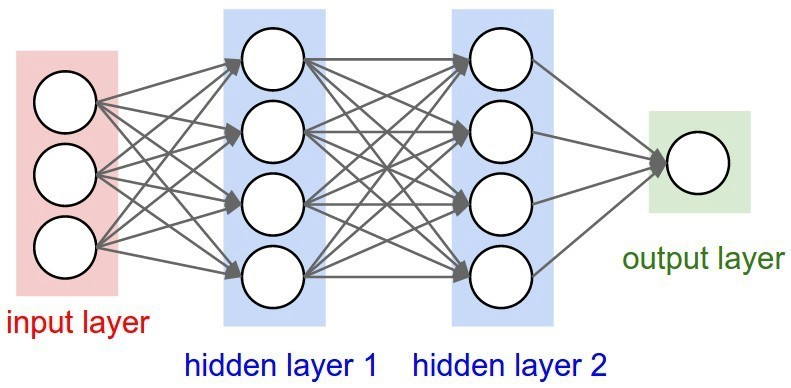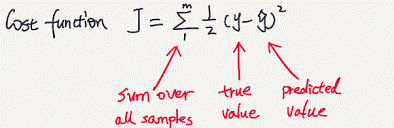# FeedForward Neural Network

`lang: en_US`

Tip: Participate in Neural Network Course Certification Quiz to test your knowledge. Scroll to the bottom and know more!

In this topic, we will discuss the feed-forward neural network briefly in the following paragraphs. There are other types of neural networks as well, and we will discuss them in their individual lessons. For now, we will call feed-forward neural networks as neural networks only.

This is what our neural network will look like:The input layer takes in data; the hidden layers perform mathematical operations, and the output layer gives an output from the network.

The circle in each layer is called 'neuron,' which, as a matter of fact, also is equivalent to the neuron in the biological brain.

The connection between each neuron (represented by an arrow) is a factor that determines how much signal from a particular neuron should go into the next layer. This factor is also called 'weight.'

Before starting the discussion, just remember these four stages of creating a neural network -

• Data Input
• Data feeding in the forward direction ( Data FeedForwarding)
• Error calculation
• Backpropagation

Now we will discuss these stages briefly.

## Data input

Consider an example of a child touching a hot stove for the first time. Before touching, he doesn't know what will happen to him once he touches the stove. So, his brain can't tell him the right course of action.

Now he touches the stove, and the heat is sensed by the nerve sensors in his hand and sends electrical impulses to the brain that it is harmful to the hand if he keeps touching the stove for a long time. So the child removes his hand in an impulse from the stove.

Now, the data that is input to our biological brain is electrical impulse, and the output is "don't touch the stove!".

Similarly, we supply data to our neural network, and it will tell the output, just like our brain.

Now note that our brain contains billions of neurons. The neural network that we are creating contains something (also called a neuron) that is comparatively very less quantity so that we may need a lot more input data.

The data which we input depends on the application that we will be performing using our neural network. We will discuss how data is given to our network when we discuss various applications in upcoming lessons.

## Data feeding in the forward direction (or Data feed forwarding)

When the data is fed to the network in the forward direction, we need to perform some mathematical operations on it so that it will give us the required results.

First, we multiply our input with 'weight' and add bias to it to get output z.

z =input*weight + bias

Weight represents the intensity of signal transfer from one neuron to another.

Bias term helps in shifting the output z up or down. This helps in better representation of output data, which will eventually help in giving excellent results.

Second, we apply an activation function on our output z to give further output a.

a=f(z)

Activation function helps in adding non-linearity properties to our network. Here, z represents the linear equation which can solve only linear problems, which is not generally the case.

## Error calculation

We will define two types of outputs:

Target - the actual output which we want

Output - the results given by our neural network.

Example: Let's say we are training our network to approximate y=x^3 line. We will supply it with inputs x and get output y. Now, using a calculator, we will calculate the target y. Next, we compare our output and target, and if they are close to each other, then our network is predicting well.

The error can be calculated using different formulas. the most commonly used is mean square error, and it is given below.## Backpropagation

Now the error we calculated above is not always low, i.e., our output is not still equal to target. Here comes in the process of teaching our network that it is wrong, and it should give results closer to the target. We do teaching with the help of backpropagation.

As the name suggests, there is backpropagation of error along with the network, and this backpropagated error will change the values of 'weights' and 'biases' so that network can give better results this time.

This process keeps on repeating until the results are excellent.

The four equations of backpropagation using gradient descent are shown below.•DJELLOULI KHALED says:

how to fixe the result of nntool when retraining pleaze

#### MATLAB Helper ®

Use Website Chat or WhatsApp at +91-8104622179